NEET  >  Faraday's Law of Electromagnetic Induction & Lenz's Law

# Faraday's Law of Electromagnetic Induction & Lenz's Law - Physics Class 12 - NEET

1. MAGNETIC FLUX

Consider a closed curve enclosing an area A (as shown in the figure). Let there be a uniform magnetic field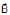in that region. The magnetic flux through the area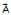is given by

...(i)

φ = BA cos θ

where θ is the angle which the vector B makes with the normal to the surface. Ifis perpendicular to, then the flux through the closed areais zero. SI unit of magnetic flux is weber (Wb).

Notes :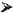Area vector is perpendicular to the surfaceFor open surface choose one direction as the area vector direction and stick to it for the whole problem.For closed surfaces outward normal is taken as area vector directionFlux is basically count of number of lines crossing a surfaceBecause magnetic field lines exists in closed loop.

Ex.1 Find flux passing through Area ?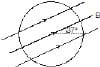Sol. Since is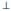to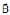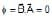2. FARADAY'S LAW OF ELECTROMAGNETIC INDUCTION

Whenever the flux of magnetic field through the area bounded by a closed conducting loop changes, an emf is produced in the loop. The emf is given by

...(ii)

where the flux of magnetic field through the area.

The emf so produced drives an electric current through the loop. If the resistance of the loop is R, then the current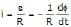...(iii)

Ex.2 A coil is placed in a constant magnetic field. The magnetic field is parallel to the plane of the coil as shown in figure. Find the emf induced in the coil.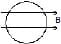Sol. f = 0 (always) since area is perpendicular to magnetic field.

Therefore, emf = 0

Ex.3 Find the emf induced in the coil shown in figure. The magnetic field is perpendicular to the plane of the coil and is constant.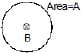Sol. f = BA (always) = const.

Therefore, emf = 0

Ex.4 Show that if the flux of magnetic induction through a coil changes from fto f2, then the charge θ that flows through the circuit of total resistance R is given by θ =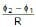, where R is the resistance of the coil.

Sol. Let f be the instantaneous flux. Then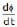is the instantaneous rate of change of flux which is equal to the magnitude of the instantaneous emf. so the current in the circuit |i| =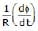, since the current is the rate of flow of charge, that is, i =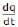θ =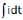or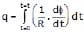where t is the time during which change takes place. but at t = 0, f = f1, and at t = t, f = f2

Therefore, θ =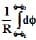=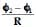3. LENZ'S LAW

The effect of the induced emf is such as to oppose the change in flux that produces it.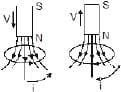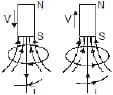(a)                (c)           (b)            (d)

In figure (a & b) as the magnet approaches the loop, the flux through the loop increases. The induced current sets up an induced magnetic field Bind whose flux opposes this change. The direction of Bind is opposite to that of external field Bext due to the magnet.

In figure (c & d) the flux through the loop decreases as the magnet moves away from the loop, the flux due to the induced magnetic field tries to maintain the flux through the loop. The direction of Bind is same as that of Bext due to magnet.

Lenz's law is closely related to the law of conservation of energy and is actually a consequence of this general law of nature. As the north pole of the magnet moves towards the loop an induced current is produced. This opposes the motion of N-pole of the bar magnet. Thus, in order to move the magnet toward the loop with a constant velocity an external force is to be applied. The work done by this external force gets transformed into electric energy, which induces current in the loop.

There is another alternative way to find the direction of current inside the loop which is described below.

Figure shows a conducting loop placed near a long, straight wire carrying a current i as shown. If the current increases continuously, then there will be an emf induced inside the loop. Due to this induced emf, an electric current is induced. To determine the direction of current inside the loop we put an arrow as shown. The right hand thumb rule shows that the normal to the loop is going into the plane. Again the same rule shows that the magnetic field at the site of the loop is also going into the plane of the diagram.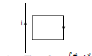Thus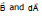are in the same direction. Therefore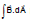is positive if i increases, the magnitude of flux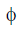increases. Since magnetic flux f is positive and its magnitude increases,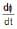is positive. Thus ε is negative and hence the current is negative. Thus the current induced is opposite, to that of arrow.

Brain Teaser

Two identical coaxial circular loops carry equal currents circulating in the same direction. What will happen to the current in each loop if the loops approach each other ?

Ex.5 Find the direction of induced current in the coil shown in figure. Magnetic field is perpendicular to the plane of coil and it is increasing with time.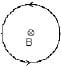Sol. Inward flux is increasing with time. To oppose it outward magnetic field should be induced. Hence current will flow in anticlockwise.

Ex.6 Figure shows a long current carrying wire and two rectangular loops moving with velocity v.

Find the direction of current in each loop.

Sol. In loop (i) no emf will be induced because there is no flux change.

In loop (ii) emf will be induced because the coil is moving in a region of decreasing magnetic field inward in direction. Therefore to oppose the flux decrease in inward direction, current will be induced such that its magnetic field will be inwards. For this direction of current should be clockwise.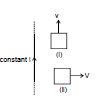4. CALCULATION OF INDUCED EMF

As we know that magnetic flux (φ) linked with a closed conducting loop = BA cos θ where B is the strength of the magnetic field, A is the magnitude of the area vector and θ is the angle between magnetic field vector and area vector.

Hence flux will be affected by change in any of them, which is discussed in the next page.

4.1 By changing the magnetic field

Ex.7 Figure shows a coil placed in decreasing magnetic field applied perpendicular to the plane of coil. The magnetic field is decreasing at a rate of 10 T/s. Find out current in magnitude and direction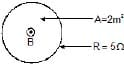Sol.= B.A

emf = A.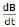= 2 × 10 = 20 v

Therefore, i = 20/5 = 4 amp. From lenz's law direction of current will be anticlockwise.

Ex.8 The magnetic flux (φ2) in a closed circuit of resistance 20 W varies with time (t) according to the equation f = 7t2 - 4t where f is in weber and t is in seconds. The magnitude of the induced current at t = 0.25 s is

(A) 25 mA (B) 0.025 mA (C) 47 mA (D) 175 mA

Sol. φ = 7t2 - 4t

⇒ Induced emf :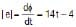⇒ Induced current :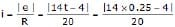(at t = 0.25 s)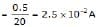Therefore, (A)

Ex.9 Consider a long infinite wire carrying a time varying current i = kt (k > 0). A circular loop of radius a and resistance R is placed with its centre at a distance d from the wire (a < < d). Find out the induced current in the loop?

Sol. Since current in the wire is continuously increasing therefore we conclude that magnetic field due to this wire in the region is also increasing.

Magnetic field B due to wire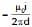going into and perpendicular to the plane of the paper

Flux through the circular loop,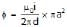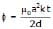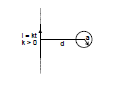Induced e.m.f. in the loop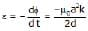Induced current in the loop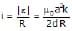Direction of induced current in the loop is anticlockwise.

Ex.10 Two concentric coplanar circular loops have diameters 20 cm and 2 m and resistance of unit length of the wire = 10-4 W/m. A time -dependent voltage V =(4 + 2.5 t) volts is applied to the larger as shown. The current in the smaller loop is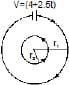Sol. r1 = 1.0 m, r2 = 10-1 m

Resistance of outer loop = 2p × 10-4 ?

Resistance of inner loop = 0.2p × 10-4 ?

Current in outer loop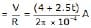or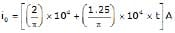Magnetic field produced at the common centre (see figure)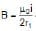or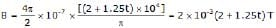Hence, flux linked with the inner loop,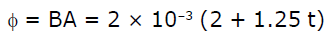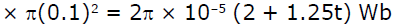Hence, the e.m.f. induced in smaller loop =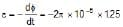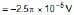The negative sign indicates that the induced e.m.f. (or current) is opposite to applied e.m.f. (or current)

Hence, the current induced in the inner (smaller) loop is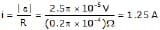Ex.11 A rectangular wire frame of length 0.2 m, is located at a distance of 5 × 10-2 m from a long straight wire carrying a current of 10 A as shown in the figure. The width of the frame = 0.05 m. The wire is in the plane of the rectangle. Find the magnetic flux through the rectangular circuit. If the current decays uniformly to 0 in 0.2 s, find the emf induced in the circuit.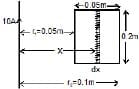Sol. A current, i = 10 A is flowing in the long straight wire. Consider a small rectangular strip (in the rectangular wire frame) of width dx at a distance x from the straight wire.

The magnetic flux at the location of the strip,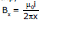The flux linked with the infinitesimally small rectangular strip

= Bx × Area of the strip = d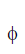x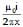l dx

where l is the length of the rectangular wire circuit

= 2 × 10-1 m

or dx = (m0 il/2p) (dx/x)

Hence, the total magnetic flux linked with the rectangular frame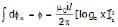or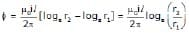Substituting values, we get= 2 × 10-7 × 10 × 2 × 10-1 × loge2

= 2.772 × 10-7 Wb

Induced e.m.f.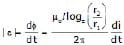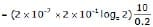= 1.386 ×10-6 V = 1.386 mV

Ex.12 Figure shows a wire frame PQSTXYZ placed in a time varying magnetic field given as B = bt, where b is a positive constant. Resistance per unit length of the wire is l. Find the current induced in the wire and draw its electrical equivalent diagram.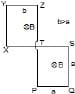Sol. Induced emf in part PQST = b a2 (in anticlockwise direction, from Lenz's Law) Similarly induced emf in part TXYZ = b b2 (in anticlockwise direction, from Lenz's Law)

Total resistance of the part PQST = l4a

Total resistance of the part PQST = l4b.

The equivalent circuit it is shown in the diagram.

writing KVL along the current flow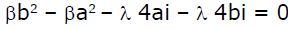i =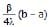Brain Teaser :

A copper ring is held horizontally and a bar magnet is dropped through the ring with its length along the axis of the ring. Will the acceleration of the falling magnet be equal to, greater than or lesser than the acceleration due to gravity ?

4.2 BY CHANGING THE AREA

Solved Examples :

Ex.13 A space is divided by the line AD into two regions. Region I is field free and the region II has a uniform magnetic field B directed into the paper. ACD is a semicircular conducting loop of radius r with centre at O, the plane of the loop being in the plane of the paper. The loop is now made to rotate with a constant angular velocity w about an axis passing through O, and perpendicular to the plane of the paper in the clockwise direction. The effective resistance of the loop is R.

(a) Obtain an expression for the magnitude of the induced current in the loop.

(b) Show the direction of the current when the loop is entering into the region II.

(c) Plot a graph between the induced emf and the time of rotation for two periods of rotation.

Sol. (a) As in time t, the arc swept by the loop in the field, i.e., region II.

A =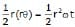So the flux linked with the rotating loop at time t,

f = BA =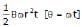and hence the induced emf in the loop,

e = -= -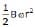= constant.

And as the resistance of the loop is R, the induced current in it,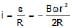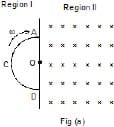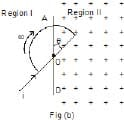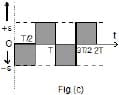(b) When the loop is entering the region II, i.e., the field figure (b), the inward flux linked with it will increase, so in accordance with Lenz's law an anticlockwise current will be induced in it.

(c) Taking induced emf to the negative when flux linked with the loop is increasing and positive when decreasing, the emf versus time graph will be, as shown in figure (c)

Ex.14 Two parallel, long, straight conductors lie on a smooth plane surface. Two other parallel conductors rest on them at right angles so as to form a square of side a initially. A uniform magnetic field B exists at right angles to the plane containing the conductors. Now they start moving out with a constant velocity (v). (a) Will the induced emf be time dependent? (b) Will the current be time dependent?

Sol. (a) Yes, φ (instantaneous flux) = B (a + 2vt)2

Therefore, e =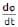= 4Bv(a + 2vt)

(b) No,

(instantaneous current) i =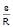Now R = 4(a + 2vt)r where r = resistance per unit length

Therefore, i =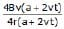=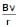(a constant)

The current will be time independent.

The document Faraday's Law of Electromagnetic Induction & Lenz's Law | Physics Class 12 - NEET is a part of the NEET Course Physics Class 12.
All you need of NEET at this link: NEET

## FAQs on Faraday's Law of Electromagnetic Induction & Lenz's Law - Physics Class 12 - NEET

 1. What is Faraday's Law of Electromagnetic Induction?Ans. Faraday's Law of Electromagnetic Induction states that a change in the magnetic field within a closed loop of wire induces an electromotive force (EMF) in the wire. This EMF leads to the generation of an electric current in the wire.
 2. How does Faraday's Law of Electromagnetic Induction work?Ans. Faraday's Law of Electromagnetic Induction is based on the principle of magnetic flux. When there is a change in the magnetic field strength or the area of the loop of wire, it induces an EMF in the wire according to the formula: EMF = -dΦ/dt, where EMF is the induced electromotive force, dΦ is the change in magnetic flux, and dt is the change in time.
 3. What is Lenz's Law?Ans. Lenz's Law is a consequence of Faraday's Law of Electromagnetic Induction. It states that the direction of the induced current in a closed loop is always such that it opposes the change that produced it. In other words, Lenz's Law ensures that the induced current creates a magnetic field that opposes the original change in magnetic flux.
 4. How does Lenz's Law relate to Faraday's Law?Ans. Lenz's Law provides a way to determine the direction of the induced current in a closed loop when there is a change in magnetic flux. It complements Faraday's Law by stating that the induced current will always oppose the change that caused it. This relationship helps to understand the behavior of electromagnetic induction and the conservation of energy.
 5. What are the practical applications of Faraday's Law and Lenz's Law?Ans. Faraday's Law and Lenz's Law have numerous practical applications. Some examples include electric power generation in power plants, transformers, induction motors, electric generators, and electromagnetic brakes. These laws are also fundamental to the functioning of devices such as speakers, microphones, magnetic levitation systems, and magnetic sensors. Overall, the laws of electromagnetic induction play a crucial role in various technologies and electrical systems.

## Physics Class 12

157 videos|466 docs|213 tests

## Physics Class 12

157 videos|466 docs|213 tests

### How to Prepare for NEET

Read our guide to prepare for NEET which is created by Toppers & the best Teachers
Signup to see your scores go up within 7 days! Learn & Practice with 1000+ FREE Notes, Videos & Tests.
10M+ students study on EduRev
Track your progress, build streaks, highlight & save important lessons and more!(Scan QR code)
Related Searches

,

,

,

,

,

,

,

,

,

,

,

,

,

,

,

,

,

,

,

,

,

;